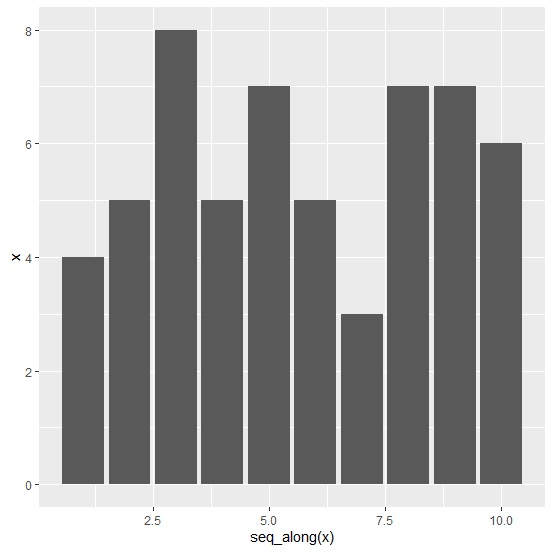# How to create a bar chart for single vector using ggplot2 in R?

To create a bar chart for single vector using ggplot2 in R, we can follow the below steps −

• First of all, create a vector and melt it using melt function of reshape2 package and save the melted data.
• Then, create the bar chart with melted data using ggplot2.

## Create the vector and melt it

Creating the vector and using melt function of reshape2 to melt the data in vector −

x<-rpois(10,5)
library(reshape2)
x_melted<-melt(x)
x_melted

On executing, the above script generates the below output(this output will vary on your system due to randomization)−

value
1 4
2 5
3 8
4 5
5 7
6 5
7 3
8 7
9 7
10 6

## Create the bar chart

Using melted data to create the bar chart and using seq_along function to display X-axis values −

x<-rpois(10,5)
library(reshape2)
x_melted<-melt(x)
library(ggplot2)
ggplot(x_melted,aes(x=seq_along(x),y=x))+geom_bar(stat="identity")

### Output